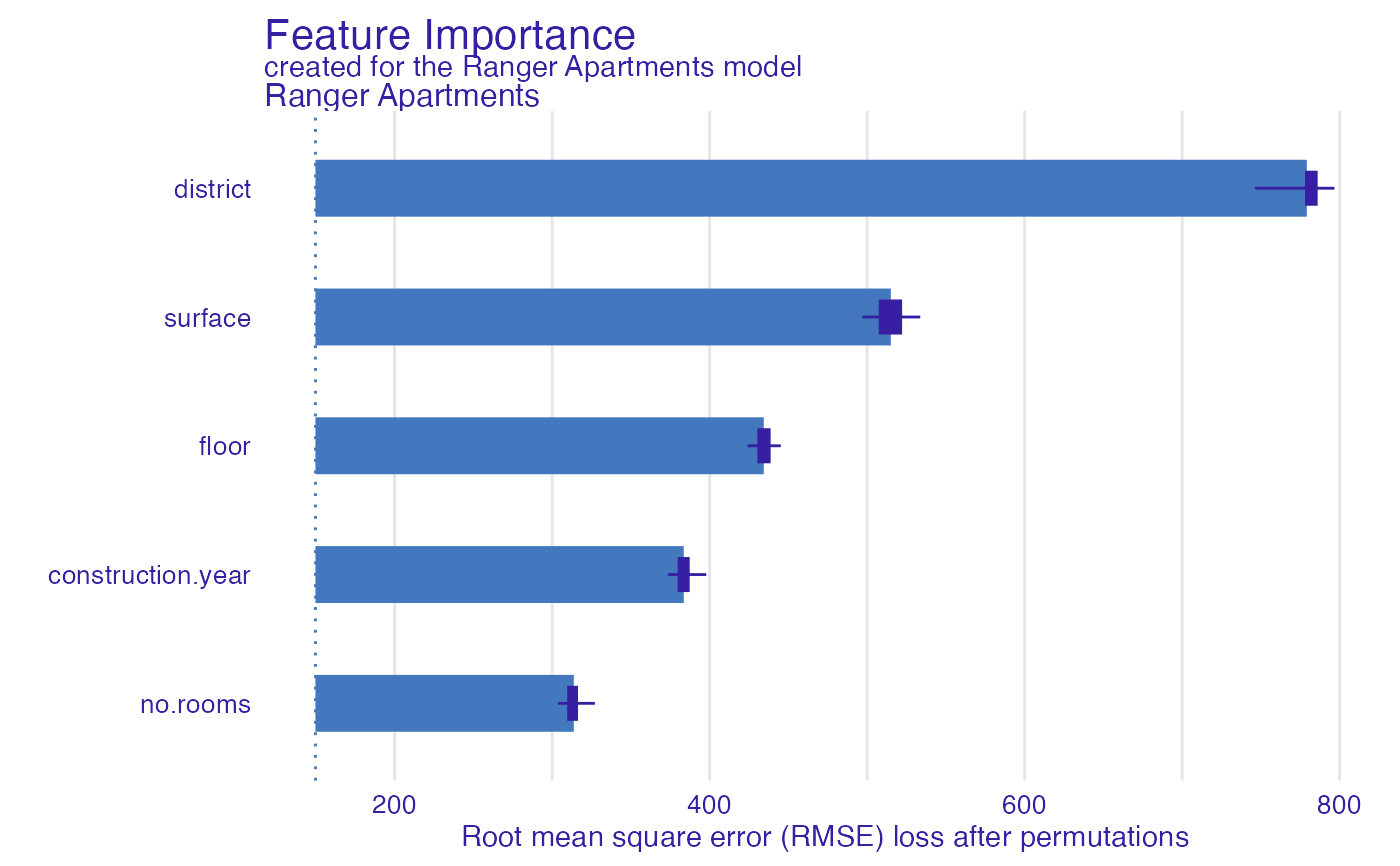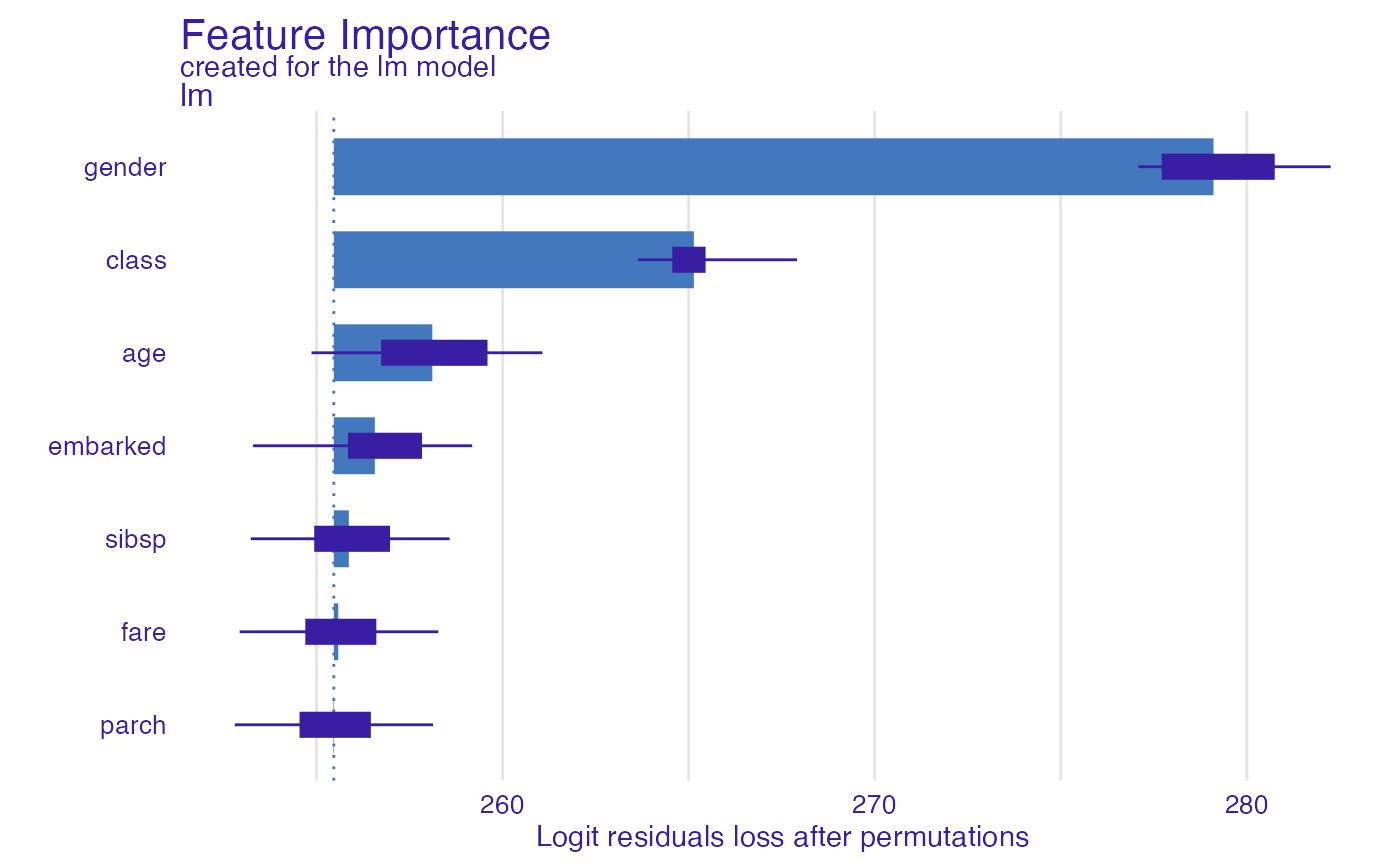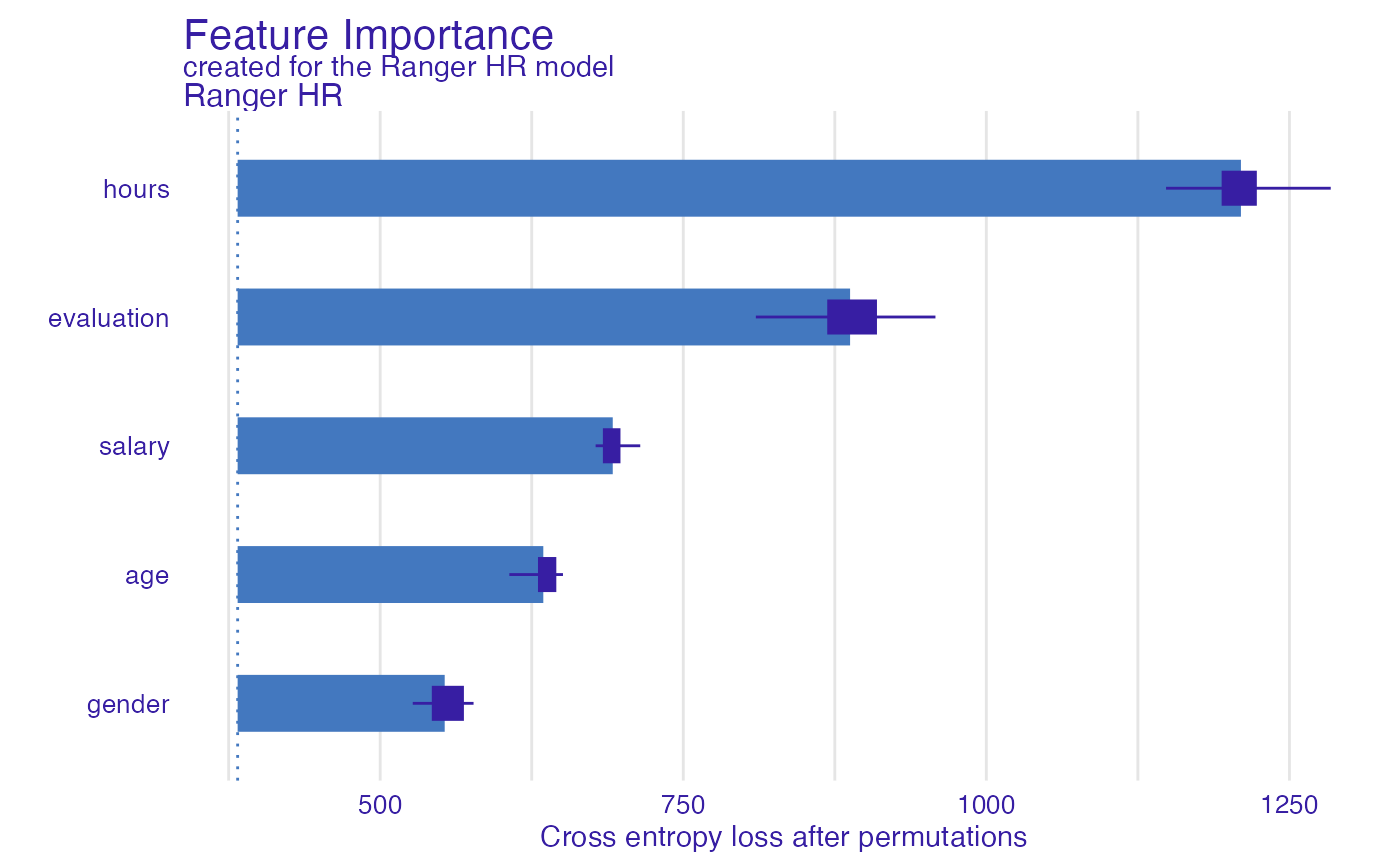From DALEX version 1.0 this function calls the feature_importance Find information how to use this function here: https://ema.drwhy.ai/featureImportance.html.

model_parts(
explainer,
loss_function = get_loss_default(explainer$model_info$type),
...,
type = "variable_importance",
N = n_sample,
n_sample = 1000
)

## Arguments

explainer

a model to be explained, preprocessed by the explain function

loss_function

a function that will be used to assess variable importance. By default it is 1-AUC for classification, cross entropy for multilabel classification and RMSE for regression. Custom, user-made loss function should accept two obligatory parameters (observed, predicted), where observed states for actual values of the target, while predicted for predicted values. If attribute loss_name is associated with function object, then it will be plotted as name of the loss function.

...

other parameters

type

character, type of transformation that should be applied for dropout loss. variable_importance and raw results raw drop lossess, ratio returns drop_loss/drop_loss_full_model while difference returns drop_loss - drop_loss_full_model

N

number of observations that should be sampled for calculation of variable importance. If NULL then variable importance will be calculated on whole dataset (no sampling).

n_sample

alias for N held for backwards compatibility. number of observations that should be sampled for calculation of variable importance.

## Value

An object of the class feature_importance. It's a data frame with calculated average response.

## Examples

# \donttest{
# regression

library("ranger")
apartments_ranger_model <- ranger(m2.price~., data = apartments, num.trees = 50)
explainer_ranger  <- explain(apartments_ranger_model, data = apartments[,-1],
y = apartments$m2.price, label = "Ranger Apartments") #> Preparation of a new explainer is initiated #> -> model label : Ranger Apartments #> -> data : 1000 rows 5 cols #> -> target variable : 1000 values #> -> predict function : yhat.ranger will be used ( default ) #> -> predicted values : No value for predict function target column. ( default ) #> -> model_info : package ranger , ver. 0.14.1 , task regression ( default ) #> -> predicted values : numerical, min = 1857.692 , mean = 3489.773 , max = 6106.26 #> -> residual function : difference between y and yhat ( default ) #> -> residuals : numerical, min = -529.2233 , mean = -2.75412 , max = 642.7184 #> A new explainer has been created! model_parts_ranger_aps <- model_parts(explainer_ranger, type = "raw") head(model_parts_ranger_aps, 8) #> variable mean_dropout_loss label #> 1 _full_model_ 149.7069 Ranger Apartments #> 2 no.rooms 313.7524 Ranger Apartments #> 3 construction.year 383.5655 Ranger Apartments #> 4 floor 434.3652 Ranger Apartments #> 5 surface 515.0340 Ranger Apartments #> 6 district 779.1501 Ranger Apartments #> 7 _baseline_ 1207.7232 Ranger Apartments plot(model_parts_ranger_aps)# binary classification titanic_glm_model <- glm(survived~., data = titanic_imputed, family = "binomial") explainer_glm_titanic <- explain(titanic_glm_model, data = titanic_imputed[,-8], y = titanic_imputed$survived)
#> Preparation of a new explainer is initiated
#>   -> model label       :  lm  (  default  )
#>   -> data              :  2207  rows  7  cols
#>   -> target variable   :  2207  values
#>   -> predict function  :  yhat.glm  will be used (  default  )
#>   -> predicted values  :  No value for predict function target column. (  default  )
#>   -> model_info        :  package stats , ver. 4.2.3 , task classification (  default  )
#>   -> predicted values  :  numerical, min =  0.008128381 , mean =  0.3221568 , max =  0.9731431
#>   -> residual function :  difference between y and yhat (  default  )
#>   -> residuals         :  numerical, min =  -0.9628583 , mean =  -2.569729e-10 , max =  0.9663346
#>   A new explainer has been created!
logit <- function(x) exp(x)/(1+exp(x))
custom_loss <- function(observed, predicted){
sum((observed - logit(predicted))^2)
}
attr(custom_loss, "loss_name") <- "Logit residuals"
model_parts_glm_titanic <- model_parts(explainer_glm_titanic, type = "raw",
loss_function = custom_loss)
#>       variable mean_dropout_loss label
#> 1 _full_model_          255.4630    lm
#> 2        parch          255.4495    lm
#> 3         fare          255.5823    lm
#> 4        sibsp          255.8662    lm
#> 5     embarked          256.5655    lm
#> 6          age          258.1096    lm
#> 7        class          265.1385    lm
#> 8       gender          279.1046    lm
plot(model_parts_glm_titanic)# multilabel classification

HR_ranger_model_HR <- ranger(status~., data = HR, num.trees = 50,
probability = TRUE)
explainer_ranger_HR  <- explain(HR_ranger_model_HR, data = HR[,-6],
y = HR\$status, label = "Ranger HR")
#> Preparation of a new explainer is initiated
#>   -> model label       :  Ranger HR
#>   -> data              :  7847  rows  5  cols
#>   -> target variable   :  7847  values
#>   -> predict function  :  yhat.ranger  will be used (  default  )
#>   -> predicted values  :  No value for predict function target column. (  default  )
#>   -> model_info        :  package ranger , ver. 0.14.1 , task multiclass (  default  )
#>   -> predicted values  :  predict function returns multiple columns:  3  (  default  )
#>   -> residual function :  difference between 1 and probability of true class (  default  )
#>   -> residuals         :  numerical, min =  0.0007937051 , mean =  0.2787164 , max =  0.8958841
#>   A new explainer has been created!
model_parts_ranger_HR <- model_parts(explainer_ranger_HR, type = "raw")
#>       variable mean_dropout_loss     label
#> 1 _full_model_          382.4245 Ranger HR
#> 2       gender          553.2105 Ranger HR
#> 3          age          634.5485 Ranger HR
#> 4       salary          691.8257 Ranger HR
#> 5   evaluation          887.5965 Ranger HR
#> 6        hours         1209.9855 Ranger HR
#> 7   _baseline_         2104.0365 Ranger HR
plot(model_parts_ranger_HR)# }